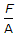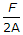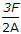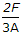# Civil Engineering - Theory of Structures - Discussion

### Discussion :: Theory of Structures - Section 1 (Q.No.35)

35.

The maximum magnitude of shear stress due to shear force F on a rectangular section of area A at the neutral axis, is

 [A].[B].[C].[D].Explanation:

No answer description available for this question.

 Kalu Charan Parida said: (Jan 16, 2015) Why it is the right answer?

 Sandeep said: (Sep 1, 2016) How is it?

 Amulya Rajbhar said: (Dec 3, 2016) (max Share stress) / (avg shear stress) = 1.5. Max shear stress at N. A. Avg Shear Stress = F/A.

 Priyanka said: (Jan 21, 2018) Anybody explain this.

 Aniruddh said: (Jan 27, 2018) At NA share stress is max. So.3F/2A is equalled to 1.5 x F/A.

 Ajay Kumar said: (Jan 24, 2019) Thanks @Amulya Rajbhar.

 Parvat Kumar said: (Aug 6, 2019) J max=FA Y/IZ. A = b(d/2-y). Y BAR = {1/2(d/2+y)+y} Z = b I = be^3/12. Jmax-6F{(d/2)^2-y^2}/bd^3. Here y = 0, Then; Jmax = 6Fd^2/4bd^3. Jmax = 3F/2A.

 Engineer Andualem Asnake said: (Aug 18, 2019) Maximum shear stress divided by average shear stress = 3/2. Hence maximum shear stress = 3/2 average shear stress. Average shear stress = F/A. Insert this equation into the 1st equation then you will get, maximum shear stress = 3F/2A.